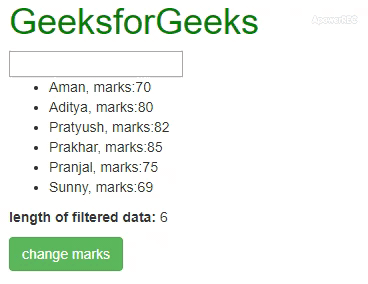Related Articles

# How to iterate over filtered (ng-repeat filter) collection of objects in AngularJS ?

• Last Updated : 30 Jan, 2020

The task is to iterate over a collection of objects already filtered by ng-repeat filters and after clicking a button, change some property of only those objects which got filtered. Suppose you have a collection of objects that were filtered by a text search within a title property on each object. Now you want to iterate over this subset of the list, after clicking an assigned button and change a property on the items that match this filter.

To iterate over a collection of objects already filtered by ng-repeat filters we use an alias expression. We create an alias of the variable used to filter the ng-repeat data. That alias variable is passed to an Angular function() when a button is clicked. Now, inside that AngularJS function, as the alias variable is containing values of only those objects which got filtered, so we iterate over each of those values. For each iteration, we change some property like in this case the marks of the filtered students by 10 and then console the values.

Syntax:

<element ng-repeat=”expression | filter: search as result”> Content… </element>
<script> result.forEach(function(student){ student.marks += 10; }); <script>

Below example illustrates the above approach:

Example: In this example we are displaying the details of students and there is a search box to search by name of the student. Now, we bind the input of that search box in the AngularJS searchName variable. SearchName variable is used to filter, we create an alias of it which is the result here and then when we click the change marks button changemarks() function is called and the result alias variable is passed as an argument. Now, inside changemarks() function we increase the marks of each filtered student by 10. So, by clicking on the change marks button we changed the marks property of only those students who were already filtered.

 ```<``html``>`` ` `<``head``>  ``    ``<``title``>``        ``Angular JS Iteration Over Filtered Data``    ````    ``<``link` `rel``=``"stylesheet"` `href``=``"https://maxcdn.bootstrapcdn.com/bootstrap/3.4.1/css/bootstrap.min.css"``>``    ``<``script` `src``=``"https://ajax.googleapis.com/ajax/libs/angularjs/1.3.14/angular.min.js"``>``    `````` ` `<``body` `style``=``"margin: 10px;"``>``    ``<``h1` `style``=``"color:green;"``> ``        ``GeeksforGeeks ``    ```` ` `    ``<``div` `ng-app``=``"mainApp"` `ng-controller``=``"studentController"``>``        ``<``input` `type``=``"text"` `ng-model``=``"searchName"``>``        ``<``br``/>`` ` `        ``<``ul``>``            ``<``li` `ng-repeat="student in students ``               ``| filter: searchName as result">``                ``{{ student.name + ', marks:' + student.marks }}``            ````        ````        ``<``p``><``b``>length of filtered data: {{ result.length }}``        ``<``buuton` `type``=``"button"` `class``=``"btn btn-success"` `                ``ng-click``=``"changemarks(result)"``>change marks``    ````  ` `    ``<``script``>``        ``var mainApp = angular.module("mainApp", []);`` ` `        ``mainApp.controller('studentController', function(\$scope) {``            ``\$scope.students = [{``                ``name: 'Aman',``                ``marks: 70``            ``}, {``                ``name: 'Aditya',``                ``marks: 80``            ``}, {``                ``name: 'Pratyush',``                ``marks: 82``            ``}, {``                ``name: 'Prakhar',``                ``marks: 85``            ``}, {``                ``name: 'Pranjal',``                ``marks: 75``            ``}, {``                ``name: 'Sunny',``                ``marks: 69``            ``}];``            ``\$scope.changemarks = function(values) {``                ``angular.forEach(values, function(value, key) {``                    ``value.marks += 10;``                    ``console.log(value.name + ' ' + value.marks);``                ``});``            ``};``        ``});``    `````` ` ``

Output:My Personal Notes arrow_drop_up